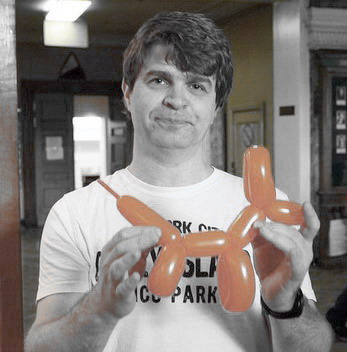## Moscow State University, Department of Physics, Acoustics Division

### Andrey V. Shaninyou can write me: a.v.shanin (round letter "a" with a tail) gmail (I am tired of spam) com
tel: +7(095) 939-3081
address: 119992, Moscow, Leninskie Gory, Moscow State University, Department of Physics

### Education

• 1988 - Moscow 57th high school
• 1994 - M.Sc. Department of Physics, Moscow State University
• 1997 - Ph.D. Department of Physics, Moscow State University

### Professional positions

• 1997-2000: Researcher, Department of Physics, Moscow State University
• 2000-2003: Senior researcher, Department of Physics, Moscow State University
• 2003-present: Reader, Department of Physics, Moscow State University

### Research interests

#### Application of multivariable complex analysis

• R.C.Assier, A.V.Shanin, Analytical continuation of two-dimensional wave fields // To appear in RSPA (2021) arXiv:2008.10673
• R.C.Assier, A.V.Shanin, Diffraction by a quarterûplane. Analytical continuation of spectral functions // QJMAM, V.72, N.1, P.51-85 (2019) (Open access)
• R.C.Assier, A.V.Shanin, Towards a multivariable Wiener-Hopf method, A mini-course in Isaac Newton Institute (Cambridge), Seminar "Bringing pure and applied analysis together via Wiener-Hopf Technique" Lecture 1 , Lecture 2 , Lecture 3 , Lecture 4 , Lecture 5

#### Lattices. Analytical approach and numerical techniques

• A.V.Shanin, A.I.Korolkov, Diffraction by a Dirichlet right angle on a discrete planar lattice // Submitted to Wave Motion arXiv preprint
• A.V.Shanin, A.I.Korolkov, Sommerfeld-type integrals for discrete diffraction problems // Wave Motion, V.97, 102606 (2020) DOI:10.1016/j.wavemoti.2020.102606 arXiv preprint
• J.Poblet-Puig, A.V.Shanin, A New Numerical Method for Solving the Acoustic Radiation Problem // Acoustical Physics, V.64, N.2, P.252-259 (2018) arXiv preprint
• J.Poblet-Puig, A.V.Shanin, A boundary algebraic formulation for plane strain elastodynamic scattering // SIAM Journal on Applied Mathematics, V.78, N.2, P.1256-1282 (2018)
• J.Poblet-Puig, V.Yu.Valyaev, A.V.Shanin, Suppression of spurious frequencies in scattering problems by means of boundary algebraic and combined field equations // J. Int. Eq. Appl., V.27, N.2, P.233-272 (2015)
• J.Poblet-Puig, V.Yu.Valyaev, A.V.Shanin, Boundary element method based on preliminary discretization Ô µ¾­ÝÓÙÕ Mathematical Models and Computer Simulations, V.6, N.2, P.172-182. (2014)

#### Waveguides and resonators

• M.A.Mironov, A.V.Shanin, A.I.Korolkov, K.S.Kniazeva, Exchange pulse related to phase synchronism in gas-plate structure // Submitted to Journ. Sound Vibr. arXiv preprint
• A.V.Shanin, K.S.Knyazeva, A.I.Korolkov Riemann surface of dispersion diagram of a multilayer acoustical waveguide Wave Motion, V.83. P.148-172. DOI: 10.1016/j.wavemoti.2018.09.010 arXiv preprint
• A.V.Shanin, Precursor wave in a layered waveguide, JASA, 141, PP. 346-356, 2017. arXiv preprint
• A.V.Shanin, A.I.Korolkov, Diffraction of a mode close to its cut-off by a transversal screen in a planar waveguide, Wave Motion, 68, PP. 218-241, 2017.
• A.I.Korolkov, S.A.Nazarov, A.V.Shanin, Stabilizing solutions at thresholds of the continuous spectrum and anomalous transmission of waves, ZAMM, 96, PP. 1245-1260, 2016.
• S.A. Nazarov, A.V. Shanin, Trapped modes in angular joints of 2D waveguides, Applicable Analysis, 93, PP. 572-582, 2014.
• E. D. Shabalina, N. V. Shirgina, and A. V. Shanin. High Frequency Modes in a Two Dimensional Rectangular Room with Windows // Acoustical Physics, (2010), Vol. 56, No. 4, pp. 525û536.
• Dorofeev M.S., Shanin A.V. Resonances in a discrete system of variable length // Acoustical Physics V. 55, N 3, pp 307-314 (2009)
• A.V. Shanin, M.S. Dorofeev, Wave modes in periodic systems of thin tubes, Proceedings of International conference ôDays on Diffraction 08ö, S.Pb, June 3-6, pp.163-168. (PDF file)
• O.V.Rudenko, A.V.Shanin, Nonlinear phenomena accompanying the development of oscillations excited in a layer of a linear dissipative medium by finite displacement of its boundary // Acoustical physics, V.46, No.3, pp.334-341 (2000).

#### Integral equation formalism for the parabolic equation of diffraction theory

• A.I.Korolkov, A.V.Shanin, A.A.Belous, Diffraction by an Elongated Body of Revolution with Impedance Boundaries: the Boundary Integral Parabolic Equation Method // Acoustical Physics, V.65, N.4, P.340-347
• A.V.Shanin, A.I.Korolkov, Diffraction by an elongated body of revolution. A boundary integral equation based on the parabolic equation, Wave Motion, V. 85, N.1, P.176-190. DOI: 10.1016/j.wavemoti.2018.10.006 arXiv preprint
• A.I.Korolkov, A.V.Shanin, The parabolic equation method and the Fresnel approximation in the application to Weinstein's problems, Journal of Mathematical Sciences, 214, PP. 302-321, 2016.
• A.I.Korolkov, A.V.Shanin, High-frequency diffraction by an impedance segment at oblique incidence // Acoustical phyiscs, V. 62, No. 6, PP. 651-658 (2016)
• A.I.Korolkov, A.V.Shanin, High-frequency plane wave diffraction by an ideal strip at oblique incidence: Parabolic equation approach // Acoustical Physics, V.62, N.4, P.405-413

#### Research on matrix factorization

• A.V.Shanin, Wiener-Hopf matrix factorization using ordinary differential equations in the commutative case arXiv preprint
Published as
A.V.Shanin, Solution of Riemann-Hilbert problem related to Wiener-Hopf factorization problem using ordinary differential equations in the commutative case // Quart. Journ. Mech. Appl. Math. Vol. 66, No 4, pp. 533-555 (2013) doi:10.1093/qjmam/hbt017.
• A.V.Shanin, An ODE-based approach to some Riemann--Hilbert problems motivated by wave diffraction arXiv preprint
• A.V.Shanin, E.M.Doubravsky, Criteria for commutative factorization of a class of algebraic matrices arXiv preprint

#### D.Sci. thesis (the defence took place on November 18, 2010)

It is entitled "New differential equations for the canonical diffraction problems" (PDF file, in Russian)

### Note

The right of distribution of each paper belongs to the publisher of corresponding Journal. However, usually I retain the right to post here a preprint or the final version of the paper, for educational purposes only. So if you want to use these materials for something else than education, please visit a library or the journal site.